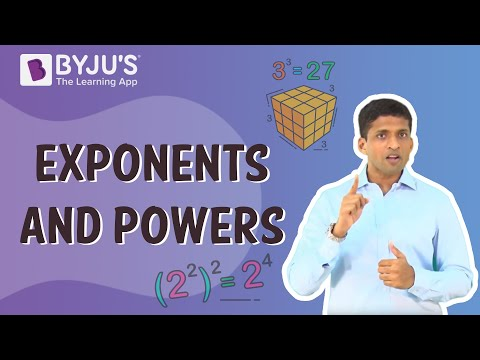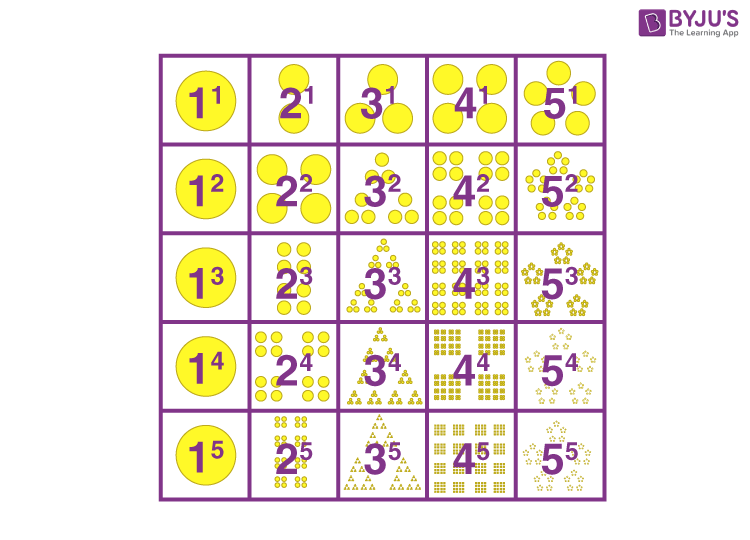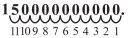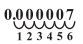# Exponents and Powers Class 8 Notes Chapter 12

According to the CBSE Syllabus 2023-24, this chapter has been renumbered as Chapter 10.

Exponents and Powers Class 8 Notes for chapter 12 given here are great study tools to boost productivity and improve overall knowledge about the topics. In the 8th standard, the concept of exponents, powers and their applications in the real world are explained clearly. This chapter helps students to build a strong foundation on the concept of exponents and powers. Solved and example problems are given here for better understanding. Students can use these notes to have a thorough revision of the entire chapter and at the same be well-equipped to write the exam.

## Introduction to Exponents and Powers

#### For more information on Powers And Exponents, watch the below video.To know more about Exponents and Powers, visit here.

### Powers and Exponents

The power of a number indicates the number of times it must be multiplied. It is written in the form ab. Where ‘b’ indicates the number of times ‘a’ needs to be multiplied to get our result. Here ‘a’ is called the base and ‘b’ is called the exponent.

For example: Consider 9³. Here the exponent ‘3’ indicates that base ‘9’ needs to be multiplied three times to get our equivalent answer which is 729.

### Powers with Negative Exponents

A negative exponent in power for any non-integer is basically a reciprocal of the power.

In simple terms, for a non-zero integer a with an exponent -b, a-b = 1/ab

## Visualising Exponents

#### For more information on Visualising Exponents And Powers, watch the below video### Visualising Powers and Exponents

Powers of numbers can easily be visualized in the form of shapes and figures. Consider the following visulization.### Expanding a Rational Number Using Powers

A given rational number can be expressed in expanded form with the help of exponents. Consider a number 1204.65. When expanded the number can be written like : 1204.65=1000+200+4+0.6+0.05=(1×10³)+(2×10²)+(0×10¹)+(4×10-1)+(5×10-2)

## Laws of Exponents

### Exponents with like Bases

Given a non-zero integer a, am×an=am+n where m and n are integers.

and am÷an=am−n where m and n are integers.

For example: 23×2= 27 + 3 = 210

and 27/2= 27−3

### Power of a Power

Given a non-zero integer a, (am)= amn, where m and n are integers.

For example: (24)= 24×3 = 212 Given a non-zero integer a,

(a)= 1 Any number to the power 0 is always 1.

### Exponents with Unlike Bases and Same Exponent

Given two non-zero integers a and b,

am×b= (a×b)m, where m is an integer.

For example: 23×5= (2×5)= 10= 1000

To know more about Exponents, visit here.

## Uses of Exponents

### Inter Conversion between Standard and Normal Forms

Very large numbers or very small numbers can be represented in the standard form with the help of exponents.

If it is a very large number like 150,000,000,000, then we need to move the decimal place towards the left. And when we do so the exponent will be positive.Since the decimal is moved 11 places till it is placed between 1 and 5, our standard form representation of the large number will be 1.5×1011

If it is a very small number like 0.000007, we need to move the decimal places to the right in order to represent the number in its standard form. When being shifted to the right, the exponent will be negative.In this case, the decimal place is moved 6 places up until it is placed after digit 7. Therefore our standard form representation will be

7×10−6

The exponents are also useful when converting the number from it’s standard form to it’s natural form.

### Comparison of Quantities Using Exponents

In order to compare two large or small quantities, we convert them to their standard exponential form and divide them.

For example : To compare the diameter of the earth and that of the sun.

Diameter of the Earth = 1.2756 × 106m

Diameter of the Sun =1.4×109m

Diameter of the Earth = 1.4×109m

1.2756 × 107m=109

So the diameter of the Sun is 109 times that of the Earth! While calculating the total or the difference between two quantities, we must first ensure that the exponents of both quantities are the same.